# Beams - Terminology and sign conventions

Many engineering structural components and machine elements take the form of a beam which bends under load. Analysis of bending and the resulting stresses and strains are the subject of several tutorials in this series.

This brief tutorial introduces the study of beams by explaining basic terms and, particularly important, the sign conventions used in subsequent tutorials.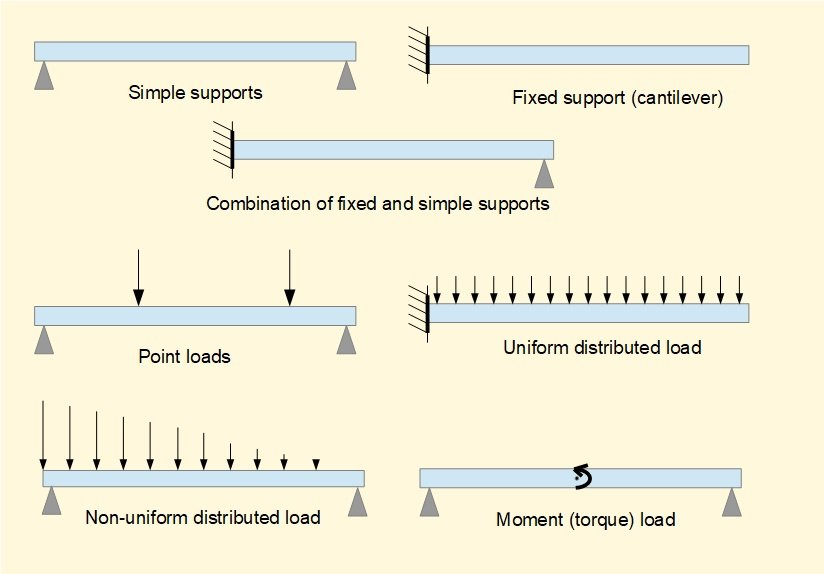We will use the following conventions for +ve and -ve directions.

This diagram shows the positive directions for x and y axes.  Thus reaction forces R1 and R2 are positive.  Loads F1 and F2 are negative.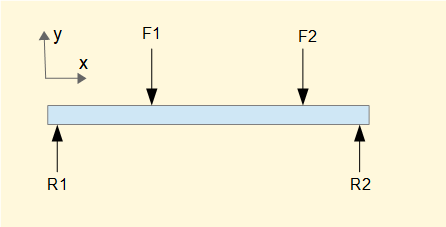In subsequent tutorials we will use extensively the conditions for static equilibrium ("sum of forces = 0" and "sum of moments  = 0").   Be aware of the following error when writing equations for the sum of forces in the example above!

It is not correct to state    R1 + R2 = - F1 - F2     (for sum of forces on the y axis = 0)

It is correct to state       R1 + R2 - F1 - F2 = 0    or      R1 + R2 = F1 + F2

The incorrect equation makes the mistake of a "double negative".  If you are prone to this error (as I am) it helps to put all terms and their signs initially on the LHS.

### Positive and negative bending

This diagram shows definitions of +ve and -ve bending.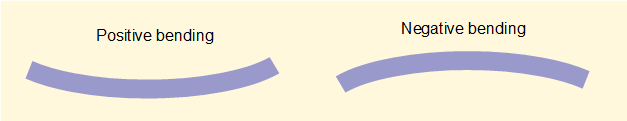We interpret bending as the consequence of bending moments  generated by loading.

To viualise this consider an element of a beam and the bending moments that produce positive or negative bending on the element.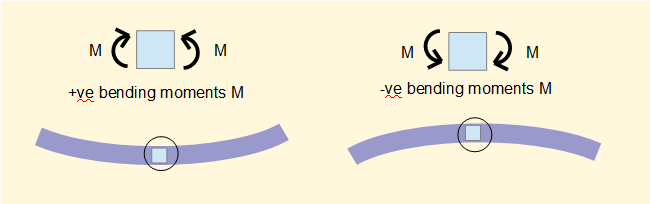In subsequent tutorials we always consider sections of beams originating at the left hand end (x = 0)  with the cut face at the right hand end of the section  Hence the sign of the bending moment M is determined by the direction of the moment shown on the right hand face of the elements above.

Note that the rotational direction of M on the right hand face of the elements above is the reverse of the normal sign convention for moment loads where clockwise moments are +ve and anti-clockwise moments are -ve

This difficulty regarding the sign of bending moments can be avoided by taking sections from the right hand end of the beam and reversing the +ve direction of the x axis.  I find this counter intuitive and prefer to reverse the sign of M.

### Positive and negative shearing forces

Vertical loads and resultant reaction forces generate vertical shearing forces in a beam. This diagram shows definitions of +ve and -ve shear.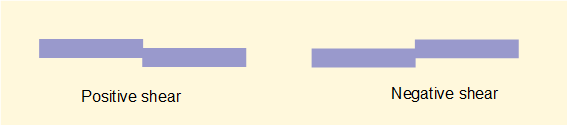To viualise this consider an element of a beam and the shearing forces that produce positive or negative shear on the element.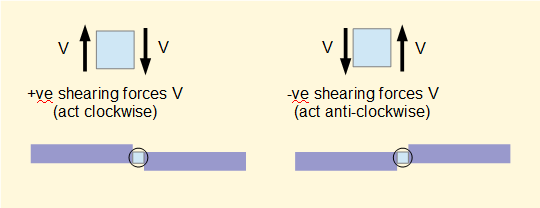In subsequent tutorials we always consider sections of beams originating at the left hand end (x = 0)  with the cut face at the right hand end of the section  Hence the sign of the shearing force V is determined by the direction of the force shown on the right hand face of the elements above.

Note that the direction of V on the right hand face of the elements above is the reverse of the sign convention for forces where upward forces are +ve

This difficulty regarding the sign of shearing forces can be avoided by reversing the sign convention for vertical forces.  I find this counter intuitive and prefer to reverse the sign of V.

Note that the sign conventions for bending moment M and shearing force V for positive and negative bending and shear as defined above are universally adopted and must not be changed.

I welcome feedback at:

### Tutorials - mechanical vibrations

###### Forced vibrations with damping

Alistair's tutorials 2019